## research papersJOURNAL OFSYNCHROTRONRADIATION
ISSN: 1600-5775
Volume 16| Part 5| September 2009| Pages 635-641

## Wave theory of focusing monochromator of synchrotron radiation

aRussian Research Center Kurchatov Institute', 123182 Moscow, Russia, and bESRF, BP 220, 38043 Grenoble, France
*Correspondence e-mail: chumakov@esrf.fr

(Received 22 November 2008; accepted 16 June 2009; online 2 July 2009)

The concept of a focusing monochromator with high energy resolution is presented. Different from conventional optical schemes, the device exploits not the angular but the spatial dispersion of synchrotron radiation. The wave theory of the monochromator is developed; it shows that the monochromator can reach an energy resolution of about 0.1 meV without significant loss of the spectral density of synchrotron radiation.

### 1. Introduction

High energy resolution is a figure of merit in many synchrotron radiation techniques. The level of radiation monochromaticity determines the accuracy of phonon spectra measured by inelastic X-ray scattering and nuclear inelastic scattering. A narrow energy bandwidth allows for an acceptable radiation load on detectors for nuclear forward scattering.

The best relative energy resolution ΔE/E served today at synchrotron radiation beamlines is about 3 × 10−8. It is determined by the quality of the best silicon crystals, in particular, by the homogeneity of their lattice constant. In absolute units, this corresponds to an energy bandwidth of about 0.5–1.0 meV for 14.412 keV radiation (Chumakov et al., 2000; Toellner, 2000).

Further improvement of the energy resolution down to 0.1 meV is one of the highly desired milestones in the development of the third-generation sources of synchrotron radiation (ESRF, APS, SPring-8). Several successful attempts to achieve an energy resolution of ∼0.1 meV have been reported (Yabashi et al., 2001; Toellner et al., 2001). However, up to now none of these approaches has developed beyond a feasibility study. The main problem of the standard approaches is a notable variance of the silicon lattice constant within a relatively large beam spot on the crystal, which results in a significant loss of spectral density of the transmitted radiation.

In this paper we consider a new type of monochromator, which we call a focusing' monochromator. Different from conventional optical schemes (Toellner, 2000; Chumakov et al., 2000; Yabashi et al., 2001; Toellner et al., 2001; Shvyd'ko, 2004), the device exploits not the angular but the spatial dispersion of synchrotron radiation.

A conventional monochromator consists of dispersing and analyzing crystals. The dispersing crystal sorts radiation of different energy over different angles. The analyser crystal picks out radiation from a narrow angular range, thus delivering radiation within an extremely narrow energy bandwidth.

In the case of the proposed focusing monochromator, the dispersion of radiation in space is provided by a lens and a downstream crystal in an asymmetric reflection. The lens focuses an X-ray beam to a narrow spot, whereas the crystal sorts out the spots of radiation of different energy over a spatial coordinate. The analyzer is a narrow slit placed in the focal plane. It picks out radiation from a narrow spatial region, thus delivering radiation within an extremely narrow energy range.

The theoretical analysis, presented below, shows that this focusing monochromator can reach very high energy resolution without significant loss of the spectral density of the transmitted radiation. From a practical point of view, the focusing monochromator operates with much smaller crystals than the traditional monochromators of comparable energy resolution. Therefore, other things being equal, this approach should be less sensitive to the possible inhomogeneity of the silicon lattice constant. Another advantage of the proposed optical scheme is that it solves two tasks at once, i.e. it prepares a (i) high-monochromatic and (ii) focused radiation beam. Finally, the focusing monochromator provides an interesting option for changing the energy bandwidth of the delivered radiation by a simple change of the slit size. Thus, researchers obtain the useful option of, for example, a fast preview of phonon spectra with moderate resolution but high count rate before longer accurate high-resolution measurements.

The theory of a conventional monochromator treats the incident radiation as a set of incoherent plane waves with defined directions and frequencies, and calculates a region of radiation reflection in the angle–frequency plane. The focusing monochromator includes a lens, a slit and crystals. It exploits spatial dispersion and spatial properties of coherent waves. Therefore, in order to analyze the operation of the focusing monochromator we develop a coherent theory, which takes into account phases of the incident radiation components and transformation of the phases owing to propagation of radiation in space, diffraction by the lens and reflection by the crystal.

### 2. Scheme of the focusing monochromator

The scheme of the focusing monochromator is shown in Fig. 1. The monochromator consists of four elements: a focusing lens, namely a parabolic refractive lens; a crystal in a symmetric reflection, which serves to keep the direction of the beam delivered by the monochromator parallel to the incident beam; a crystal in an asymmetric reflection to provide spatial dispersion of radiation components with various energies; and a slit to select the chosen energy band.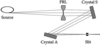Figure 1 Scheme of the focusing monochromator. Four elements are shown: the parabolic refractive lens (PRL), the crystal in a symmetric reflection (crystal S); the crystal in an asymmetric reflection (crystal A); and the slit.

As we show below, in order to obtain high energy resolution the distance z0 from the source to the lens should be as long as possible. Furthermore, the focal length f of the lens with effective aperture Ag should be chosen slightly smaller than the distance from the source. Then the lens focuses the beam almost to infinity, providing the very long focal distance zf = f/(1 − f/z0). Under these conditions the lens acts as an efficient collimator, delivering the beam with a narrow angular spread, which is important for the high energy resolution. In addition, the long focal distance zf allows one to avoid losses of intensity, because the exit angular aperture of the lens Ag/zf can be maintained small enough in order to match the narrow angular acceptance of the asymmetric reflection. The symmetric reflection does not influence the coherent properties of the beam under the considered conditions.

The asymmetric Bragg reflection should be chosen with the incidence angle larger than the exit angle (Fig. 2). Furthermore, this should be a high-order reflection, i.e. with the largest possible Bragg angle. These conditions provide highest angular dispersion, i.e. waves with various energies exit the crystal at most different angles (Souvorov et al., 1999; Shvyd'ko, 2004). The angular acceptance of the reflection in the chosen geometry is usually small. However, the narrower collimation of the incident beam allows intensity losses to be avoided.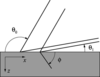Figure 2 Definition of the coordinates and angles in the case of a crystal in an asymmetric reflection.

Despite the long focal distance zf, the demagnification ratio of the proposed focusing scheme is large. This holds because, as will be shown below, the asymmetric reflection shortens the distance to the focal point, providing a small focal spot. On the other hand, owing to the high angular dispersion the radiation components with various energies are focused at sufficiently far separated points. The highest possible energy resolution is determined by the ratio of the size of the focus for a monochromatic wave to the spatial dispersion of the radiation of different energies. Increasing the slit size beyond the size of the focal spot of monochromatic radiation allows the width of the energy band delivered by the monochromator to be varied. At extreme opening of the slit, the energy bandwidth of the monochromator is equal to the bandwidth of the asymmetric reflection.

### 3. Basic formulae of coherent theory

For the sake of simplicity, we do not consider optical elements which do not influence the coherent properties of the beam; for example, the pre-monochromator and the crystal in a symmetric reflection. We assume that the radiation source is an incoherent set of point sources distributed within a region of transverse coordinates according to the Gauss law. Each point source emits a spherical wave, which is, however, confined within a finite angular region (a very narrow cone of radiation). For instance, for the undulator radiation at the ID18 beamline (Rüffer & Chumakov, 1996) at the European Synchrotron Radiation Facility (ESRF, Grenoble, France), typical parameters are 20 µm for the vertical source size S and 20 µrad for the vertical angular width of the radiation cone.

We use the paraxial approximation. It is convenient to describe an incident wave by the Kirchhoff propagator with a complex distance. We select an optical axis from the condition of highest intensity for some energy E. We consider only the diffraction plane with the coordinate x perpendicular and z along the optical axis. Because the crystals do not influence the properties of waves along the coordinate y perpendicular to the diffraction plane, we do not analyse the dependence of the radiation field along this coordinate. Propagation of the radiation field along this coordinate is described conventionally: it is divergent upstream of the lens and convergent downstream of it, if the lens has a circular aperture. In air the coordinate z is directed always along the beam and x perpendicular to the beam; in a crystal they go along the internal normal and the surface of the crystal, respectively. For convenience, we choose the directions of the x axes in various parts of the experimental scheme from the condition of acute angles between them.

We assume that the point source has a transverse coordinate xs, the distance from the source to the lens is z0, and the angular full width at half-maximum (FWHM) of the wave amplitude is a0. Then the amplitude A0(x) of the monochromatic wave component of synchrotron radiation is described by the following equation,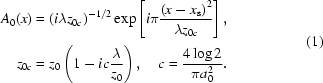Here λ is the radiation wavelength. We consider a refractive lens with biconcave shape and parabolic profile. Because of the biconcave shape (see Fig. 1), the aperture of the lens is determined by absorption (Snigirev et al., 1996). The lens is sufficiently thick that radiation is entirely absorbed beyond the lens aperture. Then, the amplitude A1(x) of the wave immediately behind the lens is expressed by the following equation,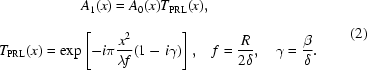Here R is the radius of curvature at the apex of the parabola, and δ and β are the real and imaginary parts of the complex refraction index of the lens material, n = 1 − δ + iβ.

Calculation of the transverse profile of the wave propagating through air is conveniently performed in reciprocal space. We introduce a coordinate of reciprocal space q which is conjugate to x, and represent the amplitude as a Fourier integral,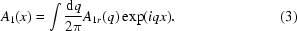Let the crystal with an asymmetric reflection be at a distance z1 downstream of the lens. In order to calculate the transverse profile of the wave in front of the crystal, it is sufficient to multiply the integrand of (3)by the Fourier image of the Kirchhoff propagator Pr(q, z1), where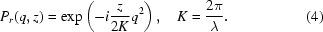Calculation of the wavefield after an asymmetric reflection by the crystal is a more complex task. First, the crystal changes the optical axis. Second, in q-space the crystal can have a finite width of reflection with a centre at q0. We need to take into account a possible rotation of the crystal by angle φ and a possible relative shift of energy δE = ΔE/E. In this case, q0 = K(−φ + δE tanθB). The highest reflectivity of the crystal corresponds to q0 = 0. The choice of the direction of rotation and the sign of the energy shift is consistent with the choice of the directions of the x and q axes. Then, in order to describe the reflection, we multiply the amplitude of the plane wave by the function R(qq0, b), where b is the asymmetry factor (see below). On the other hand, from the theory of diffraction of plane waves (Authier, 2001) it follows that the plane wave with the wavevector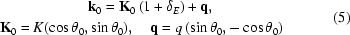is reflected to the plane wave with the wavevector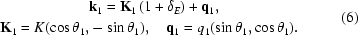Here we use the coordinate system of the crystal, and θ0 and θ1 are, respectively, the angles between the surface of the crystal and the incident or reflected beam as shown in Fig. 2. The straightforward calculation leads to the following relation between q1 and q,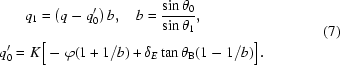We would like to emphasize that, according to (7), a polychromatic plane wave incident on the crystal at the exact Bragg angle (q = φ = 0) provides a set of monochromatic waves with various wavelengths reflected by the crystal in different directions. Introducing the variation of the exit angle Δθ1 = −q1/K, we transform (7)into the form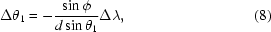where d = λ/(2sinθB) is the distance between the reflecting atomic planes, and ϕ is the angle between the reflecting plane and the surface. Equation (8)describes the `angular dispersion' (Shvyd'ko, 2004) of the initially plane wave reflected by the asymmetrically cut crystal. In general, using this effect one could immediately obtain a small energy bandwidth by selecting a narrow angular range of the exit beam with slits or a crystal analyzer. This, however, would be unpractical owing to a significant loss of photons. Instead, with the current approach, we propose to transform the angular dispersion to a spatial one, and to select a narrow energy band keeping almost the entire spectral density of the incident radiation. In the absence of asymmetry (ϕ = 0), the fan of waves with various energies does not appear (Δθ1 = 0).

In order to describe the asymmetric reflection, we need to introduce the function R, which depends on qq0 and b, and to replace the exponential factor exp(iqx) by exp(iq1x). According to (7), this leads to scaling of the transverse coordinate in the reflected beam by a factor b. Then, we obtain the amplitude A2(x) of the wave immediately behind the crystal as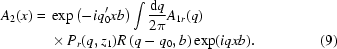Let us consider the amplitude A3(x) of the wave at some distance downstream of the crystal, in particular at the distance z2 where the wave is focused. Once again we use a known technique, i.e. we calculate the Fourier image of the wave, multiply it by the Fourier image of the Kirchhoff propagator for the distance z2 and then calculate the reverse Fourier transformation,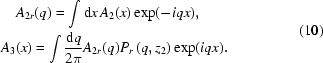Substituting (4)and (9)into (10)we obtain a triple integral including two integrals over q and one integral over x. The integral over x is equal to a δ-function, which cancels one of the integrals over q. In particular, it is convenient to keep the integral over q for the incident wave. In this case the result can be written in the form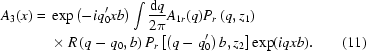Taking into account the expression (4)for the propagator, this function can be rewritten as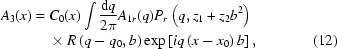where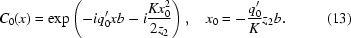In order to obtain the final expression, we need to substitute (1)into (2)and to calculate the function A1r(q). The calculation is based on the known integral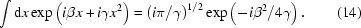We omit the calculation and write only the result,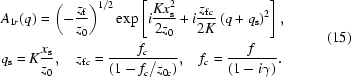Here the imaginary parts of the complex distances are neglected everywhere except for a multiplier in front of q2. This is justified if the angular size of the source as seen from the lens position is much less than the angular divergence of the incident synchrotron radiation. We substitute (15)into (12)and perform the change of variable qqqs. Then we obtain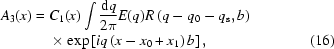where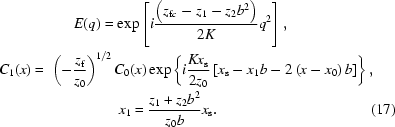Computer simulation requires an explicit expression for the function R(q, b). We consider the case of a sufficiently thick crystal, where a rear surface does not influence the reflection. In this case we obtain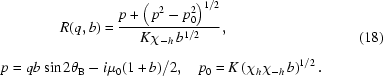Here μ0 = Im(Kχ0) is a linear absorption coefficient, χh and χh are diffraction parameters, and the square root has a positive imaginary part.

### 4. Estimation of the energy resolution

For an estimation of the energy resolution, one needs to calculate the size of the focal spot for a monochromatic wave and to compare it with the spatial dispersion of synchrotron radiation monochromatic components of various energies. Let us first consider the diffraction limit of the focal spot, i.e. the ideal case of a point source, proper crystal angular position and monochromatic wave with φ = δE = xs = 0. In this case the integrand in (16)is a Fourier image of the product of two functions; each of them has non-vanishing values only within a finite region of argument close to zero. We neglect the higher-than-linear terms in the expansion of zfc over γ and write the first function in the form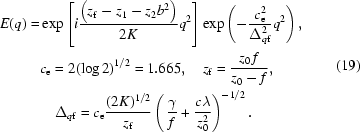Focusing of the wave takes place if the strong oscillations of the function vanish. This occurs at the distance z2 = (zfz1)/b2, which means that the asymmetric reflection shortens the distance to the focus zfz1 by a factor b2. Below we will consider a radiation field at this fixed distance. In the focal plane, E(q) is a Gauss function with a FWHM of Δqf. Let the FWHM of the amplitude of reflection by the crystal (18)be denoted as Δqc. The region of integration in (16)Δq is equal to the smaller of the two values. If Δqc > Δqf, then Δq = Δqf. Let us denote the FWHM of the integral (16)as Δx. From the theory of Fourier transformation it is known that Δxb ≃ 2π/Δq. This is an analogue of the uncertainty principle in quantum mechanics. Thus, the diffraction limit of the focus is approximately equal to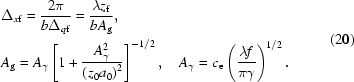Here Ag is the effective aperture and Aγ is the aperture of the lens determined by absorption (Snigirev et al., 1996). As follows from (20), the size of the beam at the lens position does not matter if it is larger than the effective aperture. On the contrary, if the effective aperture is larger than the size of the beam at the lens position, then the diffraction limit of the focus is determined by the beam size. In any event, the broadening of the focal spot originates from the limited angular region of focusing rays.

At the other extreme of Δqf > Δqc, the diffraction limit of the focal spot is determined by the finite angular range of the crystal reflection. According to (18), in this case we obtain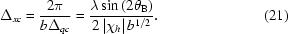In contrast to (20), the broadening of the focal spot described by (21)can be regarded as being caused by a finite region of the Bragg reflection inside the crystal bulk (Afanas'ev et al., 1971; Kohn & Kazimirov, 2007). In practice, both cases can be realised depending on the choice of the asymmetry factor b and the focal length f of the lens.

Let us consider now the spatial position of the focal spot. As follows from (16), the shift of the focus centre is determined by the value x0x1, which depends on φ, δE and xs. For a given value of the rotation angle φ the highest reflectivity of the crystal corresponds to the energy region around the relative energy δE = φ/tanθB as follows from the condition q0 = 0. Taking this into account we obtain x0 = 2φz2. Therefore we have the possibility to select the energy by rotating the crystal with a simultaneous shift of the slit.

Now we have to take into account the transverse size S of the source which leads to a broadening of the focus spot since various point sources give different focal positions. We define the size of the source projection Δs = Sz2b/z0. The expression is obtained from (17)under the condition z1 << z2b2 which is valid in the limit b >> 1. We are interested just in this limit.

The relative energy resolution ΔE of the monochromator is determined by the spatial dispersion of radiation components with various energies provided by the asymmetric reflection. As follows from (7)and (13), the relative energy shift δE corresponds to the transverse shift of the focus centre by x0 = z2(b − 1)tanθBδE. Therefore ΔE corresponds to the spatial range ΔxE = z2(b − 1)tanθBΔE. This range has to be compared with the smaller of two values, namely, of the diffraction limit of the focal spot Δx and of the size of the source projection Δs. Under the condition b >> 1, the diffraction limit, originated from a limited aperture, takes the expression Δxf = λz2b/Ag. We see that for b >> 1 all three values of ΔxE, Δs and Δxf are proportional to z2b. On the contrary, the diffraction limit, originated from a finite angular range of the crystal reflection Δxc, does not depend on the distance z2, and it decreases with increasing b. Therefore, for large z2b we can neglect broadening the focus spot by the crystal diffraction.

Therefore, if S > λz0/Ag, then the relative energy resolution is determined by the source size as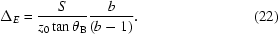In the opposite case, if S < λz0/Ag, then the energy resolution is determined by the diffraction limit as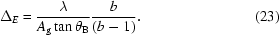In order to avoid losses in the spectral density of delivered radiation, the angular acceptance of the asymmetric reflection should be larger than the angular size of the radiation source as seen from the lens position, i.e. KS/z0 < Δqc. The angular acceptance of the asymmetric reflection is given by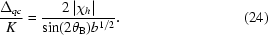Finally, we note that the performance of a multicrystal monochromator is often characterized by a DuMond diagram, which illustrates the energy and angular properties of the monochromated beam. In our case, however, this approach would not be useful because it is not relevant to focusing. Indeed, the ultimate energy resolution is described by quite different parameters, namely, by the angular width of the source size, equation (22), or by the diffraction limit of the focal spot, equation (23).

### 5. Specific example

Let us consider a specific example of the focusing monochromator with the parameters adapted for the nuclear resonance beamline ID18 at the ESRF. The beamline is optimized for the most frequently used nuclear isotope 57Fe with energy E = 14.412 keV (wavelength λ = 0.8602 Å). The vertical source size is S = 20 µm, the vertical angular FWHM of the wave amplitude is a0 = 30 µrad. We choose the source-to-lens distance z0 = 60 m and the distance between the lens and the asymmetric crystal z1 = 0.1 m. Then, the vertical FWHM of the wave amplitude at the lens is equal to a0z0 = 1.8 mm. If the effective aperture of the lens Aγ is larger than that, then according to (20)Ag = 1.8 mm. Further, the condition S > λz0/Ag is fulfilled; and the energy resolution of the monochromator is determined by the vertical size of the source according to (22). We consider a silicon crystal, reflection (975), θB = 80.39°, K|χh| = 2.408 × 10−2 µm−1. With these parameters we obtain from (22) ΔE = 5.6 × 10−8. This means that within the analyzing slit of width less than or equal to the size of the source projection for a monochromatic beam, the focusing monochromator provides synchrotron radiation with an energy bandwidth of 0.81 meV. This energy resolution is comparable with that of the best currently used multi-crystal monochromator (Chumakov et al., 2000; Toellner, 2000). Unlike the conventional devices, however, in this case the energy resolution is determined by quite different parameters, in particular by the angular size of the source. Also different from the conventional schemes, the size of the beam spot on the crystal surface is very small, less than 4 mm. Thus, the performance of the monochromator should be less affected by the possible inhomogeneity of the silicon lattice constant.

The other parameters can be chosen from practical considerations. One of the important requirements is to avoid losses of spectral density of synchrotron radiation in order to maintain the highest possible count rate in experiments. For this reason the exit angular aperture of the lens Ag/zf should not be larger than the angular width of the crystal reflection. Because the latter is very small, the focal length f has to be only slightly smaller than z0. Therefore, for the estimation of the effective lens aperture we can replace f by z0. For a Be lens (density 1.845 g cm−1) the optical parameters are δ = 1.637 × 10−6, β = 3.432 × 10−10 and γ = 2.097 × 10−4. Then the effective lens aperture is Aγ = 4.65 mm. This value is larger than a0z0. For an Al lens (density 2.694 g cm−1) we obtain δ = 2.613 × 10−6, β = 1.546 × 10−8, γ = 5.917 × 10−3 and Aγ = 0.88 mm. In this case the effective lens aperture is two times smaller than the halfwidth of the beam. Nevertheless, the condition S > λz0/Aγ is still fulfilled; and the energy resolution is determined by the vertical source size. Therefore an Al lens can also be considered for use in a focusing monochromator.

From a practical point of view, the distance z2 should not exceed the length of the experimental hutch. Therefore, it is convenient to define, first of all, this distance and to determine the rest of the parameters through z2. In such a way we find the asymmetry parameter b. The above discussed requirement to avoid losses of spectral density of synchrotron radiation can be expressed as Δxf = 2Δxc or Δqc = 2Δqf. Then using (20)and (21)we obtain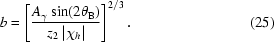Finally, we find the focal distance of the lens from the condition (19)within the approximation zf = z2b2. It is sufficient to use an approximate formula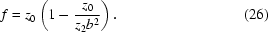Thus, for z2 = 6 m from (25)and (26)we obtain b = 28, f = 59.2 m. The slit size has to be equal to 56 µm which is technically easy to fulfil.

Fig. 3shows the distribution of the intensities of monochromatic radiation from various points of the radiation source observed at the focal plane calculated for the parameters discussed above, i.e. E = 14.412 keV, z0 = 60 m, z1 = 0.1 m, z2 = 6 m, Si (975), b = 28 and an Al lens. The curves correspond to various points of an extended Gaussian source with vertical size of 20 µm (FWHM). As follows from Fig. 3, the beam spot is significantly extended because of the finite size of the source. The reason for the small difference in the relative intensity of the curves to the left and to the right of the central peak is the change of the reflection amplitude as a function of the coordinate of the source point [equation (16)].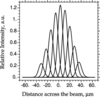Figure 3 Distribution of intensities of monochromatic radiation from several equidistant points of the radiation source observed at the focal plane. See text for details.

In order to improve further the energy resolution of the monochromator for radiation with a given energy, one can increase the distance z0, which results in a decrease of the angular size of the source. However, because the effective aperture of the lens Aγ scales only with the square root of this distance, for a large enough distance z0 the energy resolution will no longer be determined by the source size but by the diffraction limit according to formula (23). In this case one should use a Be lens because it has a larger effective aperture. According to (23), at longer distances the energy resolution is independent of the angular source size. At the crossover distance SAg/λ = 1200 m, the relative energy resolution is equal to ΔE = 3 × 10−9, which corresponds to an energy bandwidth of 0.04 meV.

Another way to improve the energy resolution is to use a reflection with the Bragg angle closer to 90°. For radiation with energy E = 14.412 keV and a Si crystal the reflection (975) is the last allowed one. The availability of high-quality single crystals other than silicon could solve the problem. For example, a sapphire (Al2O3) crystal offers useful reflections (30 0 0) with θB = 83.33° and (22 6 1) with θB = 88.02°. With z0 = 60 m, the use of these reflections would improve the energy resolution from 0.81 meV down to 0.56 meV and 0.17 meV, respectively.

For radiation of some other nuclear isotopes, reflections with θB ≲ 90° are available even with a Si crystal. For example, for the nuclear resonance in 161Dy with energy E = 25.651 keV the last allowed reflection (18 12 6) has θB = 87.44°. With z0 = 60 m, this corresponds to an energy bandwidth of 0.38 meV.

Furthermore, if there are no restrictions on the choice of the energy as, for example, in the case of inelastic X-ray scattering, then a reflection with θB = 89° would allow one to obtain a bandwidth of 0.1 meV. In this case, however, note that although the design does offer the monochromator solution, most probably it cannot be applied for the energy analysis of the scattered radiation.

### 6. Conclusion

We have analyzed the concept, the theory and several specific examples of a new type of a monochromator, i.e. a focusing monochromator. Different from conventional optical schemes, the device exploits not the angular but the spatial dispersion of synchrotron radiation. The monochromator solves two optical tasks, monochromatization and focusing, within a single optical scheme. The numerical estimations show that the monochromator can routinely reach an energy resolution of better than 1 meV and in special cases down to 0.1 meV.

In comparison with the conventional schemes, a smaller crystal size makes the focusing monochromator less sensitive to possible crystal imperfections. On the other hand, the highest achievable energy resolution depends on the effective angular size of the radiation source. This demands a high quality of other optical elements used in the beamlines, for example, the pre-monochromator.

### Acknowledgements

We thank Yu. Shvyd'ko for discussion of the optical scheme and for the numerical data on the sapphire crystal, and M. Sánchez del Río for preliminary ray-tracing calculations. The work of VGK is supported by grants RFBR 07-02-00067a and RS-4110.2008.2.

### ReferencesJOURNAL OFSYNCHROTRONRADIATION